## ML Aggarwal Class 7 Solutions for ICSE Maths Chapter 15 Visualising Solid Shapes Ex 15.1

Question 1.
Match the following shapes with their names: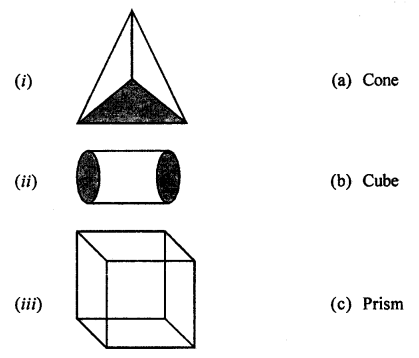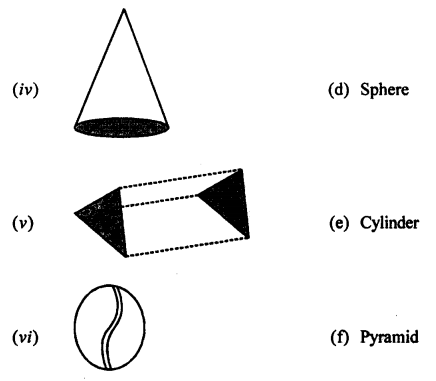Solution: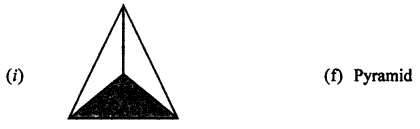Question 2.
Identify the nets which can be folded to form a cube (cut out copies of the nets and try it):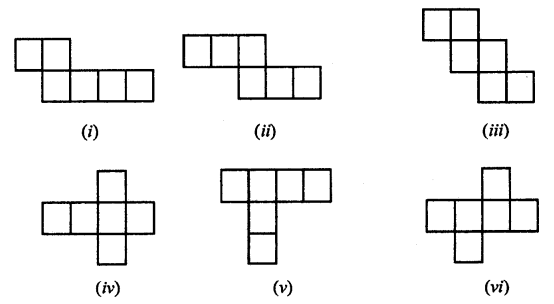Solution:
Nets (ii), (in), (iv) and (vi) form a cube.

Question 3.
Dice are cubes with dots on each face. Opposite faces of a die always have a total of sevend dots on them.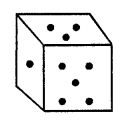Here are two nets to make dice (cubes); the numbers inserted in each square indicate the number of dots in that box.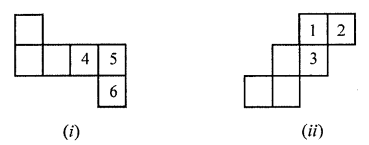Insert suitable numbers in the blanks, remembering that the number on the opposite faces should total to 7.
Solution:
The suitable numbers have been inserted in the boxes.Question 4.
Can any of the following be a net for a die? If no, explain your answer: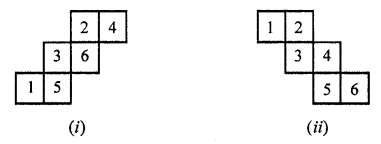Solution:
(i) Yes.
(ii) No, because one pair of opposite faces will have
1 and 4 on them whose total not 7, and another pair of
opposite faces will have 3 and 6 on them whose total is also not 7.

Question 5.
Here is an incomplete net for making a cube. Complete it in at least two different ways. Remember that a cube has six faces. How many are there in the net here? (You may use a squared paper for easy manipulation.)Solution:
The given incomplete net of a cube has been
completed in two ways as given under: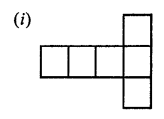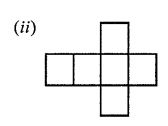(iii) Three faces out of 6 faces are given.